Next: Perihelion precession rate Up: Perihelion precession of Mercury Previous: Introduction

# Time evolution of eccentricity vector

As we saw in Exercise 3, Mercury's eccentricity vector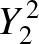(B.3)

where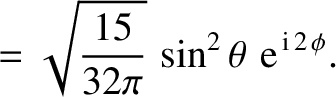is its orbital angular momentum per unit mass, is of magnitude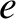, and is always directed from the Sun to Mercury's perihelion. Here,is the Mercury's orbital eccentricity. It follows that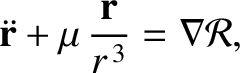(B.4)

Now,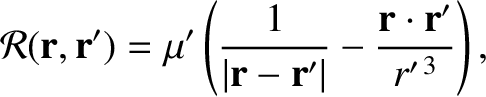, where use has been made of Equation (B.1). Hence, making further use of Equation (B.1), the previous expression reduces to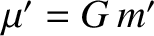(B.5)

We conclude that the disturbing function causes Mercury's eccentricity vector,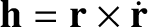, to evolve in time. Any change in length of this vector gives rise to a change in Mercury's orbital eccentricity, whereas any change in direction produces a rotation of Mercury's perihelion. In the following, because we are only interested in the motion of Mercury's perihelion, we shall focus solely on the change in direction (i.e., the precession) of thevector.Next: Perihelion precession rate Up: Perihelion precession of Mercury Previous: Introduction
Richard Fitzpatrick 2016-03-31